CAT  >  Venn Diagrams

# Venn Diagrams - Logical Reasoning (LR) and Data Interpretation (DI) - CAT

 Table of contentsWhat is a Venn Diagram?Representation of Sets with Venn DiagramsVenn Diagram SymbolsVenn Diagram for Three SetsHow to Draw a Venn Diagram?Solved ExamplesOf the 200 candidates who were interviewed for a position at call centre, 100 had a two-wheeler, 70 had a credit card and 140 had a and 140 had a mobile phone, 40 of them had both a two-wheeler and a credit card, 30 had both a credit card and a mobile phone, 60 had both a two-wheeler and a mobile phone, and 10 had all three. How many candidates had none of the three?
• a)
0
• b)
20
• c)
10
• d)
18

## What is a Venn Diagram?

• A Venn diagram (also called a set diagram or logic diagram) is a diagram that shows all possible logical relations between a finite collection of different sets.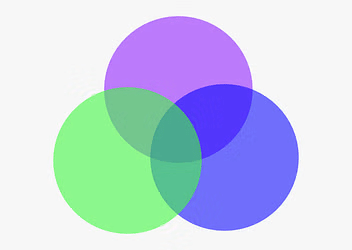Venn Diagram
• Typically overlapping shapes, usually circles, are used, and an area-proportional or scaled Venn diagram is one in which the area of the shape is proportional to the number of elements it contains.
• These diagrams represent elements as points in the plane and sets as regions inside curves. An element is in a set S just in case the corresponding point is in the region for S. They are thus a special case of Euler diagrams, which do not necessarily show all relations.
• Venn diagrams were conceived around 1880 by John Venn. They are used to teach elementary set theory, as well as illustrate simple set relationships in probability, logic, statistics, linguistics and computer science.

## Representation of Sets with Venn Diagrams

Case 1: When universal sets and a normal set have been given.
Let U be the universal set representing the sets of all natural numbers, and let A ⊆ U for A = 1,2,3,4,5
Then, by a Venn diagram, we can show as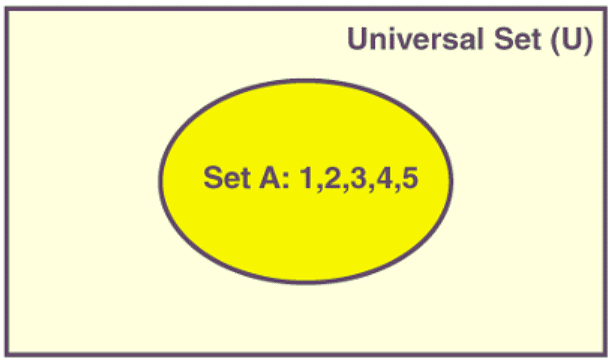Case 2: When two intersecting subsets of U are given.
To represent two intersecting subsets, A and B of U, we draw two circles, and the intersecting regions will be shown as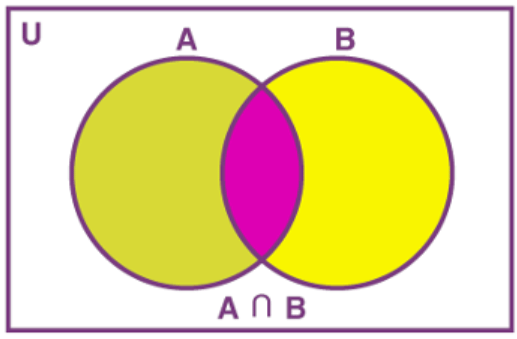Case 3: When two disjoint sets are given.
To draw two disjoint sets, we normally draw two circles which will not intersect each other within a rectangle.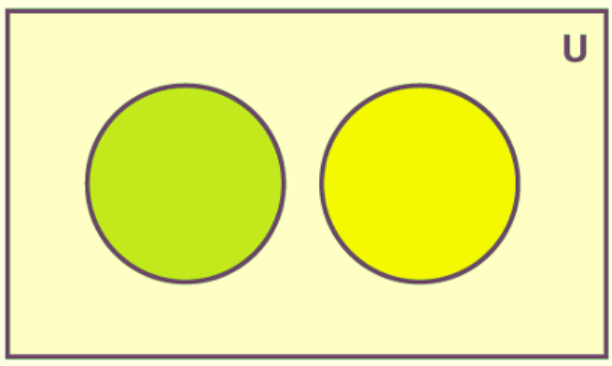Case 4: When B ⊆ A ⊆ U
In this case, we draw two concentric circles within a rectangular region.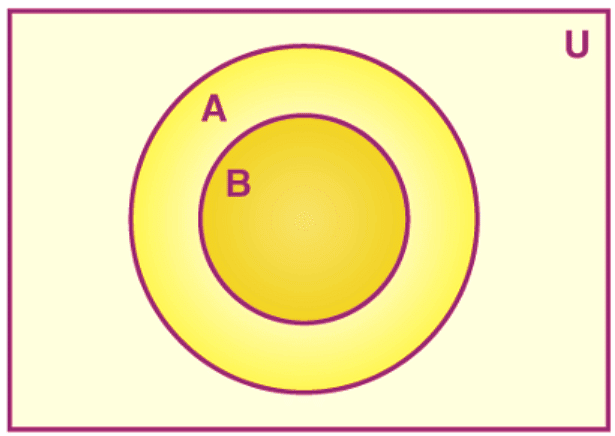Case 5: Complement of a set.
Let U be the set of natural numbers, and let A = {1, 2, 3}. Then, the complement of set A is given by
A’ = U – A = {1 2, 3, 4, 5, 6,….} – {1, 2, 3} = {4, 5, 6,…}
Then, the complement of A can be shown as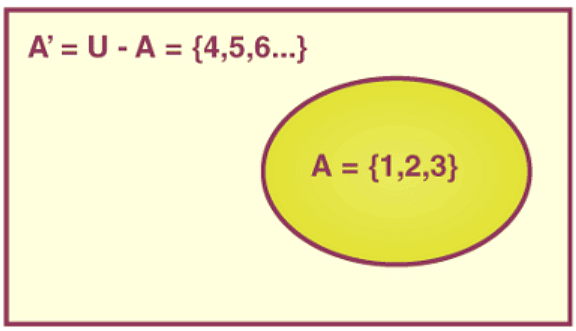## Venn Diagram Symbols

There are more than 30 Venn diagram symbols. We will learn about the three most commonly used symbols in this section. They are listed below as: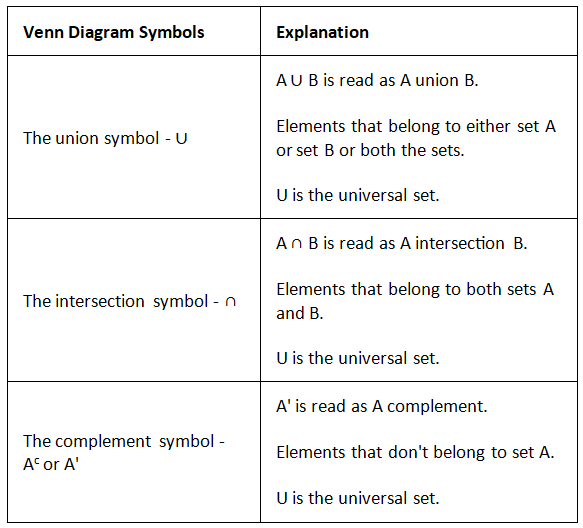Let us understand the concept and the usage of the three basic Venn diagram symbols using the image given below.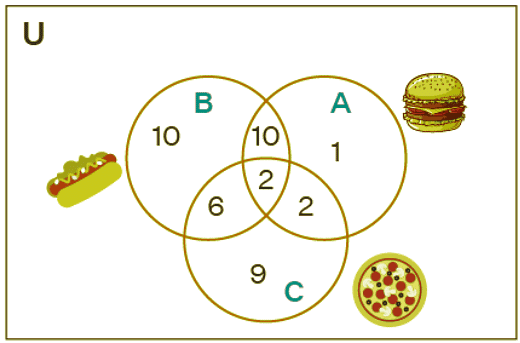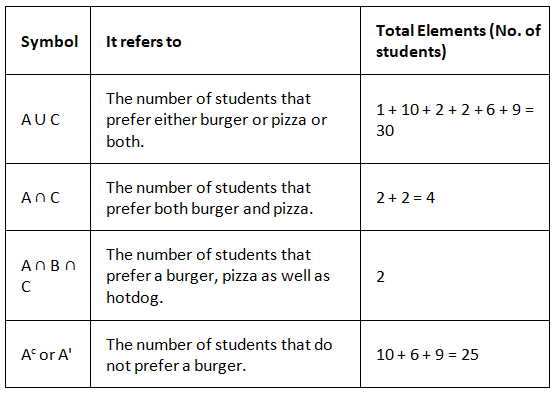## Venn Diagram for Three Sets

Three sets Venn diagram is made up of three overlapping circles and these three circles show how the elements of the three sets are related. When a Venn diagram is made of three sets, it is also called a 3-circle Venn diagram. In a Venn diagram, when all these three circles overlap, the overlapping parts contain elements that are either common to any two circles or they are common to all the three circles. Let us consider the below given example: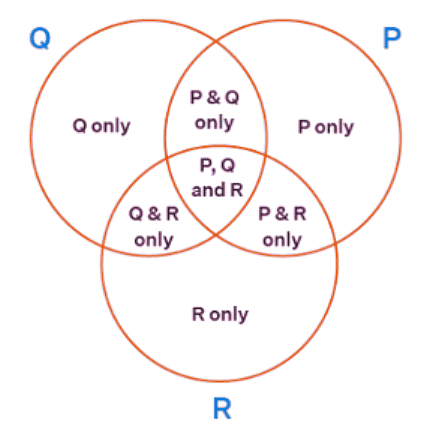Here are some important observations from the above image:

• Elements in P and Q = elements in P and Q only plus elements in P, Q, and R.
• Elements in Q and R = elements in Q and R only plus elements in P, Q, and R.
• Elements in P and R = elements in P and R only plus elements in P, Q, and R.

## How to Draw a Venn Diagram?

Venn diagrams can be drawn with unlimited circles. Since more than three becomes very complicated, we will usually consider only two or three circles in a Venn diagram. Here are the 4 easy steps to draw a Venn diagram:

• Step 1: Categorize all the items into sets.
• Step 2: Draw a rectangle and label it as per the correlation between the sets.
• Step 3: Draw the circles according to the number of categories you have.
• Step 4: Place all the items in the relevant circles.

Example: Let us draw a Venn diagram to show categories of outdoor and indoor for the following pets: Parrots, Hamsters, Cats, Rabbits, Fish, Goats, Tortoises, Horses.

• Step 1: Categorize all the items into sets (Here, its pets): Indoor pets: Cats, Hamsters, and, Parrots. Outdoor pets: Horses, Tortoises, and Goats. Both categories (outdoor and indoor): Rabbits and Fish.
• Step 2: Draw a rectangle and label it as per the correlation between the two sets. Here, let's label the rectangle as Pets.
• Step 3: Draw the circles according to the number of categories you have. There are two categories in the sample question: outdoor pets and indoor pets. So, let us draw two circles and make sure the circles overlap.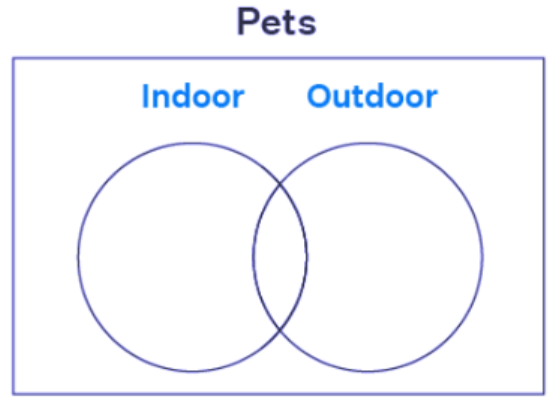• Step 4: Place all the pets in the relevant circles. If there are certain pets that fit both the categories, then place them at the intersection of sets, where the circles overlap. Rabbits and fish can be kept as indoor and outdoor pets, and hence they are placed at the intersection of both circles.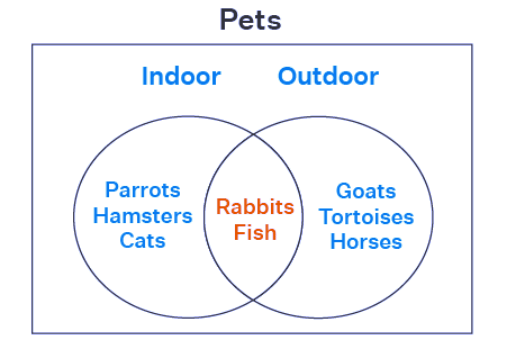• Step 5: If there is a pet that doesn't fit either the indoor or outdoor sets, then place it within the rectangle but outside the circles.

## Solved Examples

Directions for the question: Read the information given below and answer the question that follows.
Applicants for the doctoral programmes of Ambi Institute of Engineering (AIE) and Bambi Institute of Engineering (BIE) have to appear for a Common Entrance Test (CET). The test has three sections: Physics (P), Chemistry (C), and Maths (M). Among those appearing for CET, those at or above the 80th percentile in at least two sections, and at or above the 90th percentile overall, are selected for Advanced Entrance Test (AET) conducted by AIE. AET is used by AIE for final selection. For the 200 candidates who are at or above the 90th percentile overall based on CET, the following are known about their performance in CET:
1. No one is below the 80th percentile in all 3 sections.
2. 150 are at or above the 80th percentile in exactly two sections.
3. The number of candidates at or above the 80th percentile only in P is the same as the number of candidates at or above the 80th percentile only in C. The same is the number of candidates at or above the 80th percentile only in M.
4. Number of candidates below 80th percentile in P: Number of candidates below 80th percentile in C: Number of candidates below 80th percentile in M = 4:2:1.
BIE uses a different process for selection. If any candidate is appearing in the AET by AIE, BIE considers their AET score for final selection provided the candidate is at or above the 80th percentile in P. Any other candidate at or above the 80th percentile in P in CET, but who is not eligible for the AET, is required to appear in a separate test to be conducted by BIE for being considered for final selection. Altogether, there are 400 candidates this year who are at or above the 80th percentile in P.
Q1: What best can be concluded about the number of candidates sitting for the separate test for BIE who were at or above the 90th percentile overall in CET?
Sol: The number of candidates sitting for separate test for BIE who were at or above 90th percentile in CET (a) is either 3 or 10.

Q2: If the number of candidates who are at or above the 90th percentile overall and also at or above the 80th percentile in all three sections in CET is actually a multiple of 5, what is the number of candidates who are at or above the 90th percentile overall and at or above the 80th percentile in both P and M in CET?
Sol:
From the given condition, g is a multiple of 5. Hence, g = 20. The number of candidates at or above 90th percentile overall and at or above 80th percentile in both P and M = e + g = 60.

Q3: If the number of candidates who are at or above the 90th percentile overall and also at or above the 80th percentile in all three sections in CET is actually a multiple of 5, then how many candidates were shortlisted for the AET for AIE?
Sol:
In this case, g = 20. Number of candidates shortlisted for AET = d + e + f + g = 10 + 40 + 100 + 20 = 170

Q4: If the number of candidates who are at or above the 90th percentile overall and also are at or above the 80th percentile in P in CET, is more than 100, how many candidates had to sit for the separate test for BIE?
Sol:
From the given condition, the number of candidates at or above 90th percentile overall and at or above 80th percentile in P in CET = 104. The number of candidates who have to sit for separate test = 296 + 3 = 299.

Q5: In a college, 200 students are randomly selected. 140 like tea, 120 like coffee and 80 like both tea and coffee.
• How many students like only tea?
• How many students like only coffee?
• How many students like neither tea nor coffee?
• How many students like only one of tea or coffee?
• How many students like at least one of the beverages?
Sol: The given information may be represented by the following Venn diagram, where T = tea and C = coffee.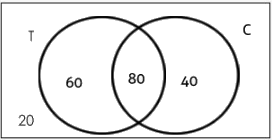• Number of students who like only tea = 60
• Number of students who like only coffee = 40
• Number of students who like neither tea nor coffee = 20
• Number of students who like only one of tea or coffee = 60 + 40 = 100
• Number of students who like at least one of tea or coffee = n (only Tea) + n (only coffee) + n (both Tea & coffee) = 60 + 40 + 80 = 180

Q6: In a survey of 500 students of a college, it was found that 49% liked watching football, 53% liked watching hockey and 62% liked watching basketball. Also, 27% liked watching football and hockey both, 29% liked watching basketball and hockey both and 28% liked watching football and basket ball both. 5% liked watching none of these games.
• How many students like watching all the three games?
• Find the ratio of number of students who like watching only football to those who like watching only hockey.
• Find the number of students who like watching only one of the three given games.
• Find the number of students who like watching at least two of the given games.
Sol: n(F) = percentage of students who like watching football = 49%
n(H) = percentage of students who like watching hockey = 53%
n(B)= percentage of students who like watching basketball = 62%
n ( F ∩ H) = 27% ; n (B ∩ H) = 29% ; n(F ∩ B) = 28%
Since 5% like watching none of the given games so, n (F ∪ H ∪ B) = 95%.
Now applying the basic formula,
95% = 49% + 53% + 62% -27% - 29% - 28% + n (F ∩ H ∩ B)
Solving, you get n (F ∩ H ∩ B) = 15%.
Now, make the Venn diagram as per the information given.
The document Venn Diagrams | Logical Reasoning (LR) and Data Interpretation (DI) - CAT is a part of the CAT Course Logical Reasoning (LR) and Data Interpretation (DI).
All you need of CAT at this link: CAT

## Logical Reasoning (LR) and Data Interpretation (DI)

125 videos|168 docs|117 tests

## FAQs on Venn Diagrams - Logical Reasoning (LR) and Data Interpretation (DI) - CAT

 1. What is a Venn diagram?A Venn diagram is a graphical representation of the relationships between different sets of data. It consists of overlapping circles or shapes, each representing a set, and the overlapping region represents the elements that belong to both sets.
 2. How can Venn diagrams be used in problem-solving?Venn diagrams can be used in problem-solving to visually represent and analyze relationships between different sets of data. They help in identifying common elements, differences, and overlaps between sets, making it easier to understand the problem and find solutions.
 3. What are the key elements of a Venn diagram?The key elements of a Venn diagram include circles or shapes representing different sets, overlapping regions representing common elements, and labels or descriptions for each set. Additionally, the diagram may include numerical values or percentages to provide further information about the data.
 4. How can Venn diagrams be used in business analysis?Venn diagrams can be used in business analysis to analyze market segments, customer behavior, and product features. They help in identifying overlapping customer needs, target markets, and competitive advantages. Venn diagrams can also be used to compare and contrast different products, services, or business strategies.
 5. Can Venn diagrams be used in academic research?Yes, Venn diagrams are widely used in academic research, especially in the fields of social sciences, statistics, and data analysis. They can be used to visualize relationships between variables, compare different groups or categories, and illustrate the intersection of multiple factors. Venn diagrams are particularly useful in presenting complex data in a clear and concise manner.

## Logical Reasoning (LR) and Data Interpretation (DI)

125 videos|168 docs|117 testsExplore Courses for CAT exam### How to Prepare for CAT

Read our guide to prepare for CAT which is created by Toppers & the best Teachers
Signup to see your scores go up within 7 days! Learn & Practice with 1000+ FREE Notes, Videos & Tests.
10M+ students study on EduRev
Track your progress, build streaks, highlight & save important lessons and more!
Related Searches

,

,

,

,

,

,

,

,

,

,

,

,

,

,

,

,

,

,

,

,

,

;